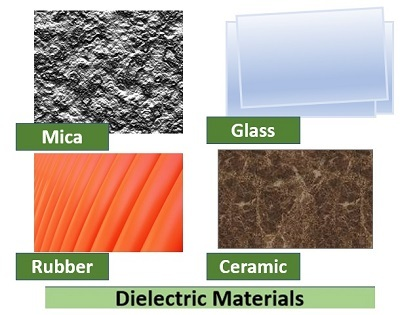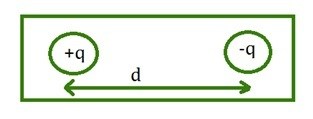# Dielectric constant of Water

## Introduction

Dielectrics materials plays a major role while studying Solid State Physics.Solid-state physics is a branch of physics that describes the physical properties of matter in a solid or solid state. Initially, the field was considered a branch of metals, and later, it expanded into a field of study of all types of solids. This field of science is at the forefront of today's modern science and technology. The sector is particularly important in electronics, integrated circuit design, and the development of various sensitive instruments. Matter can be found in three states. They are the gaseous state, solid state, and liquid state. Atoms or ions or molecules combine to form a substance. A substance is a solid, liquid, or gas depending on the atomic distance, shape, and force exerted on each other by these substances.

## Dielectric Materials

• Materials with constant electrical dipoles are called dielectric materials.

• A material is said to be dielectric if it has the property of forming a large number of induced electric dipoles when an electric field is applied to it.

• Also, this material is devoid of free electrons. The behaviour of this material changes due to the action of the electric field.

• Mostly these materials are used to store electrical energy. All electrically conductive materials can be insulators. But not all insulators are necessarily dielectric materials.

• A dielectric material is a non-metallic material with high electrical resistivity and anti-thermal resistivity. Dielectric materials play a very important role in electronic devices.

• The main characteristic of dielectric material is that when an electric field is applied, the material becomes polarized.

## Fundamental Definitions in Dielectric

### Electric Dipole

An electric dipole is a system of two charges of equal and opposite signs that are spaced apart from each other.### Dipole Moment (μ)

$$\mathrm{Dipole\:Moment =charge\times distance\:between\:the\:two\:charges}$$

Its unit is a coulomb meter.

$$\mathrm{\mu=qd\:coloumb\:meter}$$

$$\mathrm{\mu=Dipole\:Moment}$$

$$\mathrm{q=charge}$$

$$\mathrm{d=Distance\:between\:the\:two\: charges}$$

### Permittivity(ε)

The dielectric property of a medium is defined as its permittivity. It indicates the polarization nature of an object. Its unit is $\mathrm{farad\:meter^{−1}}$

For example, the value of permittivity of the vacuum is $\mathrm{\varepsilon_{0}=8.854\times 10^{−12}\:F/m}$

### Electric polarization

The displacement of the positive charge in the direction of the electric field and displacement of negative charges in the opposite direction creates a dipole in an object.

Therefore, when an electric field is applied, the phenomenon of the formation of dipoles is known as polarization.

It depends on the frequency of the applied electric field and the temperature.

## What is a Dielectric Constant?

• The dielectric constant is the ratio between the permittivity of a medium and the permittivity of a vacuum. The dielectric property of a material can be known by this value. There is no unit for this value.

$$\mathrm{\varepsilon_{r}=\frac{\varepsilon}{\varepsilon_0}=\frac{C}{C_0}}$$

$\mathrm{\varepsilon}$ =the permittivity of a medium

$\mathrm{\varepsilon_0}$ =the permittivity of vacuum

$\mathrm{C}$= the capacitance of parallel plate capacitor filled with dielectric

$\mathrm{C_0}$ =the capacitance of parallel plate capacitor filled with dielectric in vacuum

• The dielectric constant is a property that measures the electric polarization of a dielectric material.

$$\mathrm{\varepsilon_r=\frac{E_0}{E_0-E_p}}$$

$\mathrm{E_0}$ =applied electric field

$\mathrm{E_p}$ =polarised electric field

• If there is more polarization in a material then $\mathrm{E_p}$ will be more. So $\mathrm{\varepsilon_r}$ will be higher in that case.

## Dielectric Constant of Water

• Water is the most abundant naturally occurring compound of hydrogen. 70% of the earth's surface is covered by seawater, which is the main source of water.

• Water is a colourless liquid that evaporates easily. Water has unique properties in the condensed state due to intermolecular hydrogen bonding.

• The dielectric constant of water is 78.39. The high boiling point of water is due to hydrogen bonding in water.

## Conclusion

Matter can be found in three states. They are gaseous state, solid state and liquid state. Atoms or ions or molecules combine to form a substance. A material is said to be dielectric if it has the property of forming a large number of induced electric dipoles when an electric field is applied to it.

An electric dipole is a system of two charges of equal and opposite signs that are spaced apart from each other. The dielectric property of a medium is defined as its permittivity. It indicates the polarization nature of an object. The dielectric constant of water is 78.39. The high boiling point of water is due to hydrogen bonding in water.

## FAQs

Q1. What is Dielectric Strength?

Ans. Dielectric strength is the minimum voltage required per unit thickness of a material to cause a dielectric breakdown. As the temperature, humidity, and lifetime of a material increase, the dielectric strength of the material decreases. Its unit is $\mathrm{ volt\:m^{−1}}$

Q2. Define Electric Susceptibility

Ans. Placing a dielectric material in an electric field will produce an electric polarization. Hence the polarization vector P is directly proportional to the electric field E. That is,

$$\mathrm{polarization\:vector\:P\varpropto\:Electric \:field\:E}$$

$$\mathrm{P\:\varpropto\:E}$$

$$\mathrm{P=\chi^{E}}$$

$$\mathrm{\therefore\:\chi=\frac{P}{E}}$$

Therefore, electric susceptibility is the ratio between the polarization vector and the applied electric field.

Q3. What is dielectric loss?

Ans.

• When an alternating electric field is applied to a dielectric medium, most of the energy of the electric field is absorbed by the medium.

• Thus, the molecules in the medium heat up as energy is absorbed. Hence absorbed energy is lost as heat energy. This energy loss is called dielectric loss.

• In general, the dielectric loss is due to the imaginary part of the complex dielectric constant.

Q4. Define dielectric breakdown

Ans.

• Dielectric materials are those with high resistance and negative thermal resistance coefficient.

• In a dielectric material, as the field strength increases, the charge displacement also increases.

• Above a certain variable field value, electrical breakdown occurs in the dielectric material and current flows. There are many causes of dielectric breakdown.

Q5. What is Space-charge polarization?

Ans. When an electric field is applied to a dielectric material having two different or multiple phases, charges are separated and collected in electrodes. This phenomenon is called space-charge polarization. This type of polarization occurs because the resistance of one phase is much higher than the other.

Q6. Explain the thermal properties of dielectric materials

Ans.

• Dielectric materials can also be used in high-temperature applications. These materials should be capable of withstanding high temperatures without affecting other properties.

• Dielectric materials can also be used in high-temperature applications. These materials should be capable of withstanding high temperatures without affecting other properties.

Q7. Explain the chemical properties of insulating materials

Ans.

• When insulating materials are placed along with conductors, the properties of the insulating materials change.

• For example, chemical reactions take place when rubber is added to copper. To prevent chemical reactions, the surface of the copper should be coated with tin.

• A chemically resistant material can be considered the best insulating material.

Updated on: 06-Apr-2023

1K+ Views